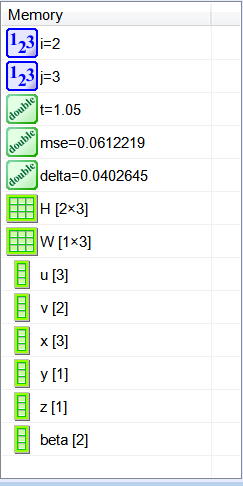# Back Propagation

## Learning Rate

The learning rate is parameter that represents how fast an ANN is being trained. The learning rate range is from 0 to 1. When the learning rate is closed to 1, the ANN may learn quickly, however, the quality of the training may be compromised.

## Back Propagation

The method of back propagation is used to train ANN. The method begins by computing the ANN output with the current weights values. At each iteration, the weights are updated in a way that the actual network output is closer and closer to the target. The method has five steps:
1. Find the output value for each output neuron.
2. Compute the mse.
3. Compute the delta for each output neuron using the formula shown below.
4. Compute the beta for each hidden neuron using the formula shown below.
5. Update the weights using the formula shown below.
Note that the formulas apply only when the activation function is the z=logsig(z).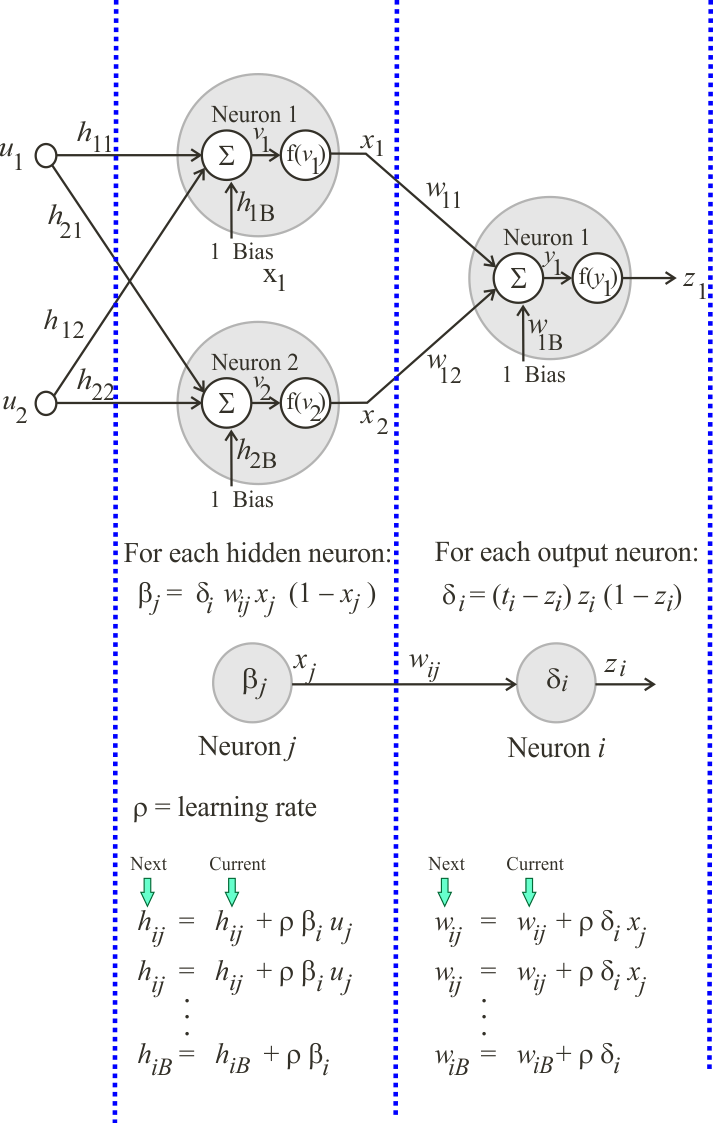Problem 1 Create a Neural Lab project called FindOutput. Find the values of: x1, x2, y1 and z1, suppose the activation function is logsig(x).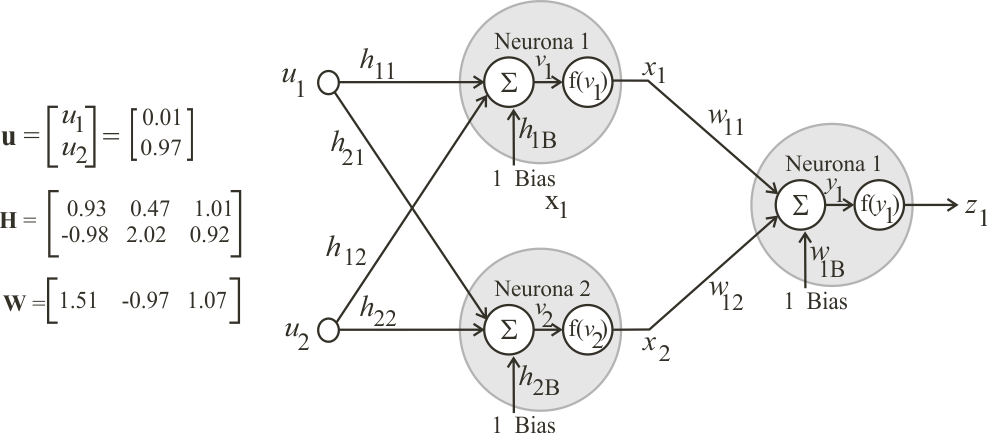FindOutput.lab Vector u; u.Create(... Matrix H; H.Create(... Vector v = ... Vector x = ... Matrix W; W.Create(... Vector y = ... Vector z = ...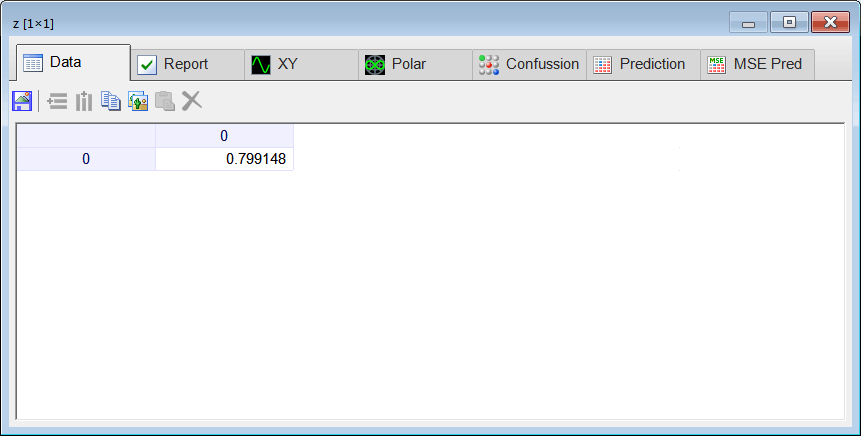Problem 2 Compute the mse when the target of the ANN is 1.05.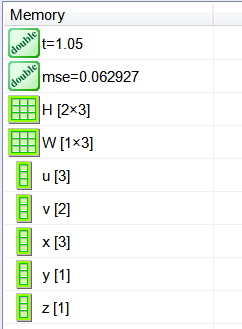Problem 3 Compute the delta of the output neuron.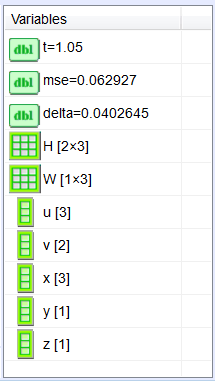Problem 4 Compute the beta for each neuron in the hidden layer. In this specific case, there is only one delta, thus, you must use i = 1 to compute beta.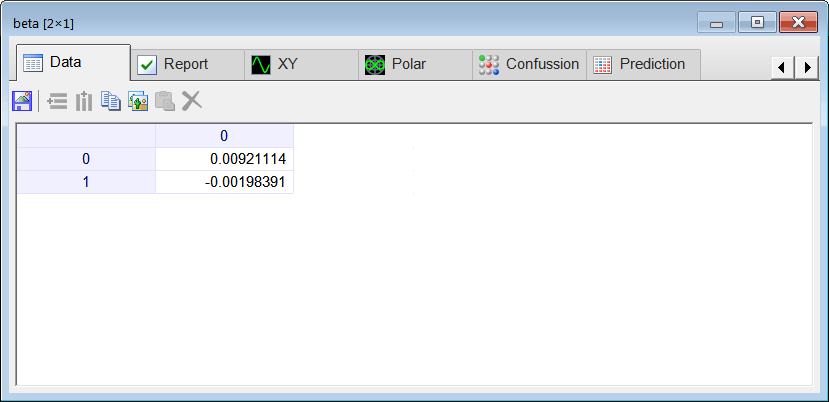Problem 5 Compute the new weights: H and W. Suppose that the learning rate is 0.2.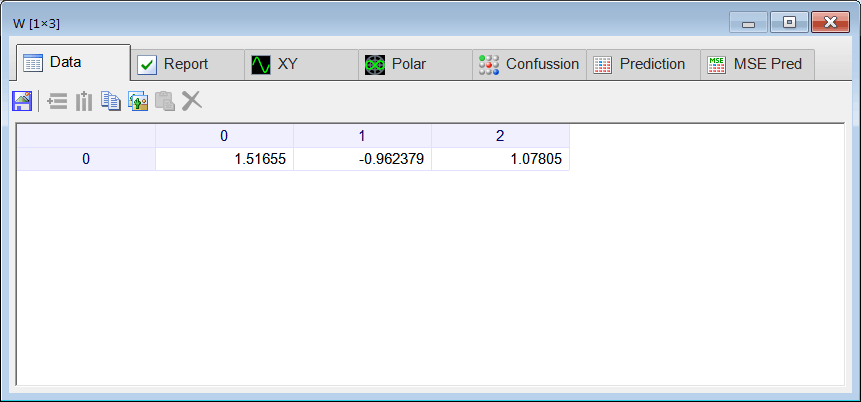Problem 6 For the new weights, compute the values of: x1, x2, y1 and z1.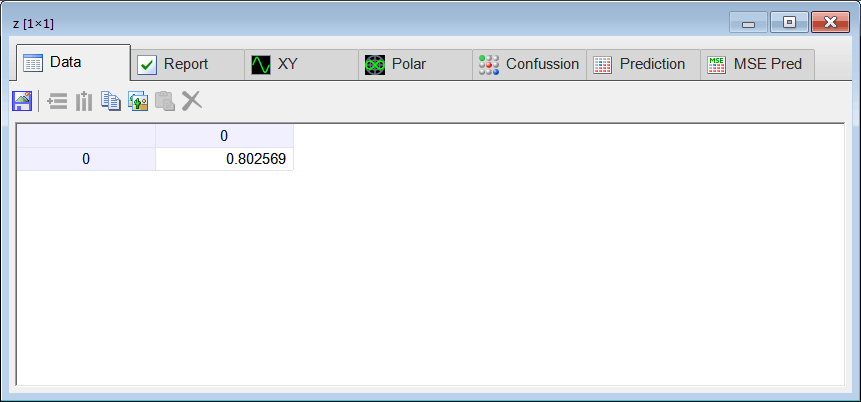Problem 7 For the new weights, compute the mse when the target of the ANN is 1.05. The new mse must be smaller than the previous one.